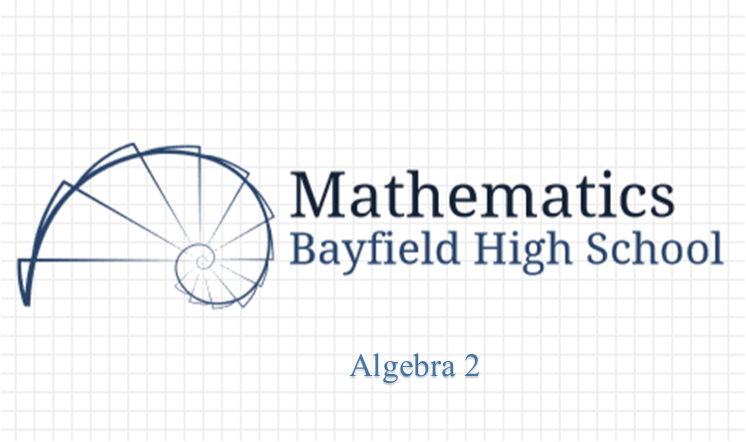# Algebra 2

HomeDo you need a GRAPHING CALCULATOR to complete your homework???  The following two links are online graphing calculators.

https://www.desmos.com/calculator

http://my.hrw.com/math06_07/nsmedia/tools/Graph_Calculator/graphCalc.html

Course Description

Algebra 2 is the study of functions and the translations of the graphs of those functions. The course extends the content of Algebra I and provides further development of the concept of a function. Topics include: (1) relations, functions, equations and inequalities; (2) conic sections; (3) polynomials; (4) algebraic fractions; (5) logarithmic and exponential functions; (6) sequences and series; (7) counting principles and probability; and (8) circular trigonometry. A scientific calculator (such as the TI-30XS) is required. Prerequisite: Geometry

Class Announcements

Supplies Needed Students will need a graph paper composition notebook, loose-leaf paper and 3 ring binder, pencil, and scientific or graphing (preferred) calculator for each class day. Composition notebooks can be purchased from the math department for \$2 and calculators can be rented from the department on a first come first serve basis (\$5 for the year or \$0.25 per class period for scientific and \$20/year or \$0.50/class period for graphing calculator). We highly encourage students to buy their own because we have a limited number. The math department will have a limited number for purchase.